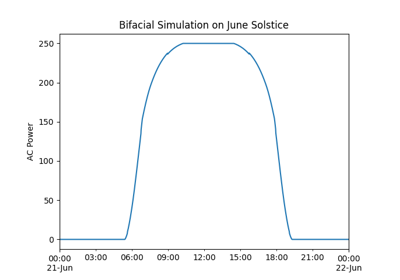Run the model starting with effective irradiance in the plane of array.

Parameters

data (DataFrame, or list or tuple of DataFrame) –

Required column is `'effective_irradiance'`. Optional columns include `'cell_temperature'`, `'module_temperature'` and `'poa_global'`.

If the ModelChain’s PVSystem has multiple arrays, data must be a list or tuple with the same length and order as the PVsystem’s Arrays. Each element of data provides the irradiance and weather for the corresponding array.

Returns

self

Raises
• ValueError – If the number of DataFrames in data is different than the number of Arrays in the PVSystem.

• ValueError – If the DataFrames in data have different indexes.

Notes

Optional `data` columns `'cell_temperature'`, `'module_temperature'` and `'poa_global'` are used for determining cell temperature.

• If optional column `'cell_temperature'` is present, these values are used and temperature_model is ignored.

• If optional column `'module_temperature'` is preset, temperature_model must be `'sapm'`.

• Otherwise, cell temperature is calculated using temperature_model.

The cell temperature models require plane-of-array irradiance as input. If optional column `'poa_global'` is present, these data are used. If `'poa_global'` is not present, `'effective_irradiance'` is used.

Assigns attributes to results: `times`, `weather`, `total_irrad`, `effective_irradiance`, `cell_temperature`, `dc`, `ac`, `losses`, `diode_params` (if dc_model is a single diode model).

## Examples using `pvlib.modelchain.ModelChain.run_model_from_effective_irradiance`#Bifacial Modeling - modelchain

Bifacial Modeling - modelchain